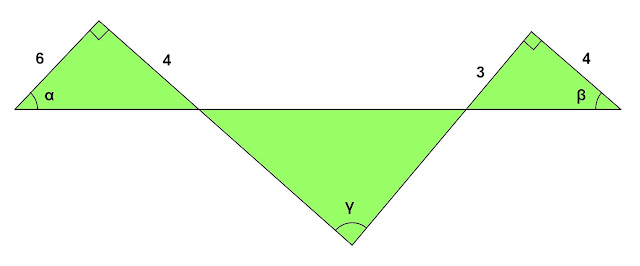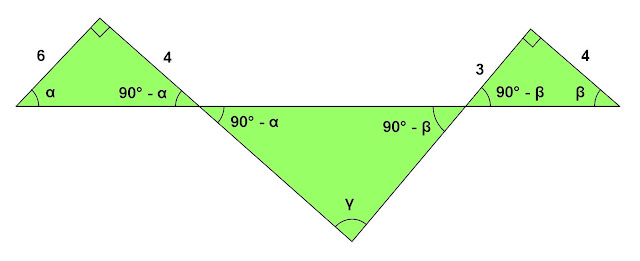## Sunday, July 28, 2013

### Three Triangles - Three Unknown Angles

Category: Trigonometry, Plane Geometry

"Published in Suisun City, California, USA"

Refer to the figure. Show that α + β = γ, and find tan γ.Photo by Math Principles in Everyday Life

Solution:

Referring to the given figure abovePhoto by Math Principles in Everyday Life

Since the two small triangles are right triangles, then we can get the other angle of two triangles as followsPhoto by Math Principles in Everyday Life

The two vertices of a big triangle are vertical angles with the other two triangles. The sum of the interior angles of a big triangle is calculated as follows

Therefore,

Consider again the figure abovePhoto by Math Principles in Everyday Life

We can get the tangent of α and β as follows

If

Take tangent on both sides of the equation, we have

Substitute the values of tan α and tan β to the above equation

Therefore,